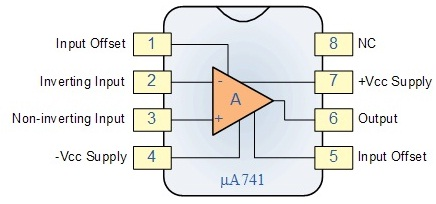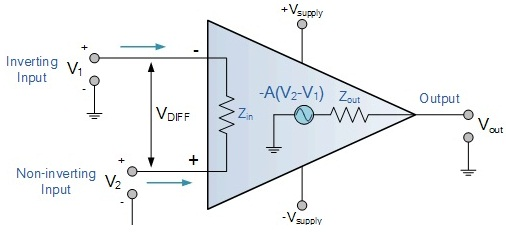Opamp Half Wave Rectifier

Objective

To design and study precision half wave rectifier using Op-amp.

Introduction

A rectifier is an electrical device that converts alternating current (AC), which periodically reverses direction, to direct current (DC), which flows in only one direction. The process is known as rectification. The precision rectifier, also known as a Superdiode, is a configuration obtained with an operational amplifier in order to have a circuit behave like an ideal diode and rectifier. It is useful for high-precision signal processing.

Rectifier circuits are used in the design of power supply circuits. In such applications, the voltage being rectified is usually much greater than the diode voltage drop, rendering the exact value of the diode drop unimportant to the proper operation of the rectifier.

Other applications exist, however, where this is not the case. For example, in instrumentation applications, the signal to be rectified can be of very small amplitude, say 0.1 V, making it impossible to employ the conventional rectifier circuits. Also the need arises for very precise transfer characteristics.

There are many applications for precision rectifiers, and most are suitable for use in audio circuits. A half wave precision rectifier is implemented using an op amp, and includes the diode in the feedback loop. This effectively cancels the forward voltage drop of the diode, so very low level signals (well below the diode's forward voltage) can still be rectified with minimal error.

• The circuit has some serious limitations. The main one is speed. It will not work well with high frequency signals.
• For a low frequency positive input signal, 100% negative feedback is applied when the diode conducts. The forward voltage is effectively removed by the feedback, and the inverting input follows the positive half of the input signal almost perfectly.
• When the input signal becomes negative, the op amp has no feedback at all, so the output pin of the op amp swings negative as far as it can.
• When the input signal becomes positive again, the op amp's output voltage will take a finite time to swing back to zero, then to forward bias the diode and produce an output. This time is determined by the op amp's slew rate, and even a very fast op amp will be limited to low frequencies.

• Ideal Op-amp: The figure below shows an example of an ideal operational amplifier. The main part in an amplifier is the dependent voltage source that increases in relation to the voltage drop across Rin, thus amplifying the voltage difference between V + and V -. Many uses have been found for Op-amp and an ideal Op-amp seeks to characterize the physical phenomena that make Op-amps useful.Fig.1 Op-amp 741Fig.2 Ideal Op-amp

Vs+ and Vs- are not connected to the circuit within the Op-amp because they power the dependent voltage source’s circuit. These are notable, however, because they determine the maximum voltage the dependent voltage source can output. For any input voltage the ideal Op-amp has,
a. Infinite open-loop gain
b. Infinite bandwidth
c. Infinite input impedance
d. Zero offset voltage
e. Infinite slew rate
f. Zero output impedance and
g. Zero noise